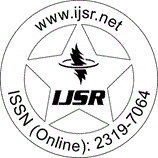International Journal of Science and Research (IJSR)
Call for Papers | Fully Refereed | Open Access | Double Blind Peer Reviewed

Research Paper | Mathematics | Iraq | Volume 6 Issue 6, June 2017

# Efficient Iterative Method for Initial and Boundary Value Problems Appear in Engineering and Applied Sciences

Majeed Ahmed AL-Jawary | Mustafa Mahmood Azeez

Abstract: The main aim and contribution of the current paper is to implement a semi-analytical iterative method proposed by Temimi and Ansari namely (TAM) to solve the Riccati, pantograph and elastic beam deformation equations, which appeared in models of various problems in engineering and applied sciences. The exact solutions are obtained for Riccati, Pantograph equations and an approximate solution for beam equation. The convergence of the TAM is investigated for the three problems. In general, the accuracy of our result for beam equation is better than those of Homotopy perturbation method (HPM) and Variational Iteration Method (VIM). The software used for the terms calculation in iterative process was MATHEMATICA 10.

Keywords: Differential equations, Riccati equation, Pantograph equation, Elastic beam deformation equations, Iterative method

Edition: Volume 6 Issue 6, June 2017,

Pages: 529 - 538